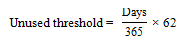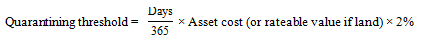# Application of rules to part-years

(Clause 19)

### Summary of proposed amendments

The various calculations necessary under the new rules will be adjusted on a pro-rata basis if the asset is held for only part of the year.

### Application date

The amendments will apply from the beginning of the 2013–14 income year.

### Key features

Section DG 22 contains formulas for calculating the 62-day non-use threshold and a 2 percent quarantining threshold when the asset is held only for part of the year. The formulas are:

• The first is for calculating the 62-day unused threshold under section DG 2:• The second is for calculating the 2 percent quarantining threshold under section DG 15:Days in the formulas are the number of days in the income year in which the asset meets the definition of an asset under these rules.

Example

If an aircraft is purchased 100 days into an income year at a cost of \$100,000 and for the rest of the income year that aircraft is used both to earn income and used privately, the total amount of unused days that are needed to satisfy the unused time threshold in section DG 2 is 45 days (265/365 x 62). The gross income required to exceed the 2 percent loss ring-fencing threshold in section DG 8 is \$1,452 (265/365 x \$100,000 x 2%).

Section DG 22 also provides the rules for calculating a company’s debt value and a company’s interest expenditure when the asset is held for only part of the year:

• The debt value of the company is defined in section DG 11 as the average outstanding amount that gives rise to the interest payable by the company, measured by reference to the amounts outstanding at the start and at the end of an income year. However, if the asset was acquired or disposed of during the year, the average outstanding debt value is measured from the time the company acquired the asset or from the time the asset was disposed of.
• The interest expenditure of a company is also measured from the time the company acquired the asset or from the time the asset was disposed of.

### Background

The proposed 62-day unused threshold and the 2 percent quarantining threshold are designed to work on an income-year basis. The two formulas in section DG 22 appropriately pro-rate the thresholds in years when the asset has been purchased or sold, and therefore only used for part of the year.

No pro-rating applies to the \$1,000 gross income threshold below which the person who has the asset can treat it as being outside the tax system. This threshold is set for compliance cost reasons, so it is appropriate that it applies at the same level regardless of how short a period in the income year the person has the asset.